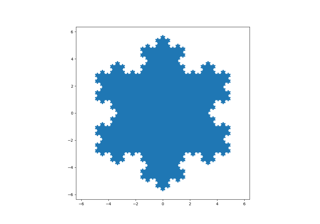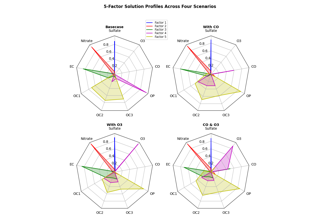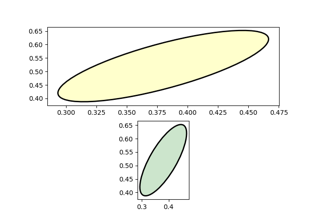# matplotlib.axes.Axes.fill#

Axes.fill(*args, data=None, **kwargs)[source]#

Plot filled polygons.

Parameters:
*argssequence of x, y, [color]

Each polygon is defined by the lists of x and y positions of its nodes, optionally followed by a color specifier. See `matplotlib.colors` for supported color specifiers. The standard color cycle is used for polygons without a color specifier.

You can plot multiple polygons by providing multiple x, y, [color] groups.

For example, each of the following is legal:

```ax.fill(x, y)                    # a polygon with default color
ax.fill(x, y, "b")               # a blue polygon
ax.fill(x, y, x2, y2)            # two polygons
ax.fill(x, y, "b", x2, y2, "r")  # a blue and a red polygon
```
dataindexable object, optional

An object with labelled data. If given, provide the label names to plot in x and y, e.g.:

```ax.fill("time", "signal",
data={"time": [0, 1, 2], "signal": [0, 1, 0]})
```
Returns:
list of `Polygon`
Other Parameters:
**kwargs`Polygon` properties

Notes

Use `fill_between()` if you would like to fill the region between two curves.

## Examples using `matplotlib.axes.Axes.fill`#Filled polygon

Filled polygonRadar chart (aka spider or star chart)

Radar chart (aka spider or star chart)Ellipse with units

Ellipse with units